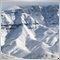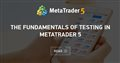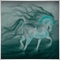# MT4 Expert Advisor places same order twice28

Hi Everyone. I have recently built a Moving Average cross over EA.

My issue is that it sometimes places the same order twice and I cannot find the reason why. I only experience this issue when live trading (1M Charts) and the problem doesn't show up on Strategy Tester

Please note that I am a new self taught EA coder so there might be bad habits so feel free to bend my ear on those. If there is a similar topic on the forum, please post a link. Thanks in advance.

See part of code attached.

```//+------------------------------------------------------------------+
//|                                              PipCrawler_2020.mq4 |
//|                                                     MVC Projects |
//|                                      https://www.mvcprojects.com |
//+------------------------------------------------------------------+
#property version   "1.00"
#property strict
//+------------------------------------------------------------------+
// Parameters Definitions                                            |
//+------------------------------------------------------------------+
//datetime ExpiryDate= D'2020.08.23 00:00';
/*int ClientAcc               = 12345;*/ // Client account number
input double LotSizeA         = 0.01; // 1 Micro lots (0.01x100)

extern int ExpMA50            = 50;  // The period for the 50 MA
extern int ExpMA100           = 100; // The period for the 100 MA
extern int ExpMA200           = 200; // The period for the 200 MA

input int Slippage            = 3;   // The slippage value
extern int MagicNumber        = 34;  // EA identifier

//+------------------------------------------------------------------+
//| Global Variables                                                 |
//+------------------------------------------------------------------+
extern int SellTicket;
extern double UsePoint;
extern int UseSlippage;

extern int SellTicket_A;
//+------------------------------------------------------------------+
//| Expert initialization function                                   |
//+------------------------------------------------------------------+
int OnInit()
{
return(INIT_SUCCEEDED);
}
//+------------------------------------------------------------------+
//| Expert deinitialization function                                 |
//+------------------------------------------------------------------+
void OnDeinit(const int reason)
{
//---
}
//+------------------------------------------------------------------+
//| Expert tick function                                             |
//+------------------------------------------------------------------+
void OnTick()
{
//+-------------------------+
//| Define string variables |
//+-------------------------+
string MA_Cross    ="";
//+---------------------------------------------------------------------------------------+
//Moving average calculations                                                             |
//+---------------------------------------------------------------------------------------+
double ExpMA50_1   = iMA(Symbol(),Period(), ExpMA50,  0, MODE_EMA, PRICE_CLOSE, 1);//Candle 1 MA Calculations
double ExpMA100_1  = iMA(Symbol(),Period(), ExpMA100, 0, MODE_EMA, PRICE_CLOSE, 1);
double ExpMA200_1  = iMA(Symbol(),Period(), ExpMA200, 0, MODE_EMA, PRICE_CLOSE, 1);

//+---------------------------------------------------------------------------------------+
double ExpMA50_2   = iMA(Symbol(),Period(),ExpMA50,  0, MODE_EMA, PRICE_CLOSE, 2);//Candle 2 MA Calculations
double ExpMA100_2  = iMA(Symbol(),Period(),ExpMA100, 0, MODE_EMA, PRICE_CLOSE, 2);
double ExpMA200_2  = iMA(Symbol(),Period(),ExpMA200, 0, MODE_EMA, PRICE_CLOSE, 2);

//+-----------------------------------------------------------------------------------------------------------------------+
int digits=Digits();

for(int i=OrdersTotal()-1;i>=0; i--) //Count all orders
&& (OrderMagicNumber()==3400||OrderMagicNumber()==3401))//Order Selection

double TrailingStopLoss = 0;

if (ExpMA100_1 > ExpMA200_1)
if (ExpMA100_2 < ExpMA200_2)
{
MA_Cross="CrossOver";
}
//+------------------------------------------+
{
if(MA_Cross=="CrossOver")
{
if(Close>Open)
{
{
}
}
}
}
//+-------------------------------------A-Trailing Stop------------------------------------------------------+
for (int i=OrdersTotal()-1; i>=0; i--)
{
{
if(OrderSymbol()==Symbol())//Check if order symbol belongs to current chart
{
{
if(OrderMagicNumber() == 3400)
{
if(OrderStopLoss()==0 || OrderStopLoss() < A_BuyStopLoss)//Check Stoploss
{
{
if(!TrailingStopLoss)
Print("Trailing Stop Failed. Error code=",GetLastError());
else Print("Order successfully modified.");
}
}
}
}
}
}
}
//+-----------------------------------------------------------------------------------------------------------------------+
// SELL ORDERS                                                                                                            |
for(int i=OrdersTotal()-1;i>=0; i--) //Count all orders
if(OrderSelect(i,SELECT_BY_POS,MODE_TRADES) && OrderSymbol()==Symbol() && OrderType()== OP_SELL
&& (OrderMagicNumber()==3420||OrderMagicNumber()==3421))

double A_Sell = NormalizeDouble(Bid-(1000*Point()),digits);//TakeProfit

//+----------Sell Crossover Signal 1 ------------------+
if (ExpMA100_1 < ExpMA200_1)
if (ExpMA100_2 > ExpMA200_2)
{
MA_Cross="CrossUnder";//Main Large Moving Average Cross
}
//+------------------------------------------+
if(sell_trades ==0) //If no open sell position
{
if (MA_Cross=="CrossUnder")
{
if (Close<Open)
{
SellTicket_A=OrderSend(Symbol(),OP_SELL,LotSizeA,Bid,Slippage,0,A_Sell,"SellOrder",3420,0,Red);
{
if(SellTicket_A < 0)
Print("SellOrderSend failed. Error Code =",GetLastError());
else Print("SellOrderSend Successful.");
}
}
}
}
//+-------------------------------------A-Trailing Stop------------------------------------------------------+
for (int i=OrdersTotal()-1; i>=0; i--)
{
{
if(OrderSymbol()==Symbol())//Check if order symbol belongs to current chart
{
if (OrderType()==OP_SELL)
{
if(OrderMagicNumber() == 3420)
{
if(OrderStopLoss()==0 || OrderStopLoss() > A_SellStopLoss)//Check Stoploss
{
TrailingStopLoss = OrderModify(OrderTicket(),OrderOpenPrice(),A_SellStopLoss,OrderTakeProfit(),0,Red);
{
if(!TrailingStopLoss)
Print("Trailing Stop Failed Error code=",GetLastError());
else Print("Order successfully modified.");
}
}
}
}
}
}
}

}//Void OnTick parantheses
```The Fundamentals of Testing in MetaTrader 5
• www.mql5.com
The idea of ​​automated trading is appealing by the fact that the trading robot can work non-stop for 24 hours a day, seven days a week. The robot does not get tired, doubtful or scared, it's is totally free from any psychological problems. It is sufficient enough to clearly formalize the trading rules and implement them in the algorithms, and...Moderator
25474

`if(sell_trades ==0) //If no open sell position`

Where do you calculate the value of sell_trades?28

Keith Watford:

Where do you calculate the value of sell_trades?

```//+-----------------------------------------------------------------------------------------------------------------------+
// SELL ORDERS                                                                                                            |
for(int i=OrdersTotal()-1;i>=0; i--) //Count all orders
if(OrderSelect(i,SELECT_BY_POS,MODE_TRADES) && OrderSymbol()==Symbol() && OrderType()== OP_SELL
&& (OrderMagicNumber()==3420||OrderMagicNumber()==3421))6118

Make sure that the same expert is not running at the same currency more than once. Even they had same magic number...Moderator
25474

thiezo:28

Keith Watford:

I have since updated my post to reflect most of my code. This Expert Advisor is intended for multi trade conditions hence the different Magic Number for each trade.

```//+------------------------------------------------------------------+
//|                                              PipCrawler_2020.mq4 |
//|                                                     MVC Projects |
//|                                      https://www.mvcprojects.com |
//+------------------------------------------------------------------+
#property version   "1.00"
#property strict
//+------------------------------------------------------------------+
// Parameters Definitions                                            |
//+------------------------------------------------------------------+
//datetime ExpiryDate= D'2020.08.23 00:00';
/*int ClientAcc               = 12345;*/ // Client account number
input double LotSizeA         = 0.01; // 1 Micro lots (0.01x100)

extern int ExpMA50            = 50;  // The period for the 50 MA
extern int ExpMA100           = 100; // The period for the 100 MA
extern int ExpMA200           = 200; // The period for the 200 MA

input int Slippage            = 3;   // The slippage value
extern int MagicNumber        = 34;  // EA identifier

//+------------------------------------------------------------------+
//| Global Variables                                                 |
//+------------------------------------------------------------------+
extern int SellTicket;
extern double UsePoint;
extern int UseSlippage;

extern int SellTicket_A;

//+------------------------------------------------------------------+
//| Expert initialization function                                   |
//+------------------------------------------------------------------+
int OnInit()
{
return(INIT_SUCCEEDED);
}
//+------------------------------------------------------------------+
//| Expert deinitialization function                                 |
//+------------------------------------------------------------------+
void OnDeinit(const int reason)
{
//---
}
//+------------------------------------------------------------------+
//| Expert tick function                                             |
//+------------------------------------------------------------------+
void OnTick()
{

//+-------------------------+
//| Define string variables |
//+-------------------------+
string MA_Cross    ="";
//+---------------------------------------------------------------------------------------+
//Moving average calculations                                                             |
//+---------------------------------------------------------------------------------------+
double ExpMA50_1   = iMA(Symbol(),Period(), ExpMA50,  0, MODE_EMA, PRICE_CLOSE, 1);//Candle 1 MA Calculations
double ExpMA100_1  = iMA(Symbol(),Period(), ExpMA100, 0, MODE_EMA, PRICE_CLOSE, 1);
double ExpMA200_1  = iMA(Symbol(),Period(), ExpMA200, 0, MODE_EMA, PRICE_CLOSE, 1);

//+---------------------------------------------------------------------------------------+
double ExpMA50_2   = iMA(Symbol(),Period(),ExpMA50,  0, MODE_EMA, PRICE_CLOSE, 2);//Candle 2 MA Calculations
double ExpMA100_2  = iMA(Symbol(),Period(),ExpMA100, 0, MODE_EMA, PRICE_CLOSE, 2);
double ExpMA200_2  = iMA(Symbol(),Period(),ExpMA200, 0, MODE_EMA, PRICE_CLOSE, 2);

//+-----------------------------------------------------------------------------------------------------------------------+
int digits=Digits();

for(int i=OrdersTotal()-1;i>=0; i--) //Count all orders
&& (OrderMagicNumber()==3400||OrderMagicNumber()==3401))//Order Selection

double TrailingStopLoss = 0;

if (ExpMA100_1 > ExpMA200_1)
if (ExpMA100_2 < ExpMA200_2)
{
MA_Cross="CrossOver";
}
//+------------------------------------------+
{
if(MA_Cross=="CrossOver")
{
if(Close>Open)
{
{
}
}
}
}
//+-------------------------------------A-Trailing Stop------------------------------------------------------+
for (int i=OrdersTotal()-1; i>=0; i--)
{
{
if(OrderSymbol()==Symbol())//Check if order symbol belongs to current chart
{
{
if(OrderMagicNumber() == 3400)
{
if(OrderStopLoss()==0 || OrderStopLoss() < A_BuyStopLoss)//Check Stoploss
{
{
if(!TrailingStopLoss)
Print("Trailing Stop Failed. Error code=",GetLastError());
else Print("Order successfully modified.");
}
}
}
}
}
}
}
//+-----------------------------------------------------------------------------------------------------------------------+
// SELL ORDERS                                                                                                            |
for(int i=OrdersTotal()-1;i>=0; i--) //Count all orders
if(OrderSelect(i,SELECT_BY_POS,MODE_TRADES) && OrderSymbol()==Symbol() && OrderType()== OP_SELL
&& (OrderMagicNumber()==3420||OrderMagicNumber()==3421))

double A_Sell = NormalizeDouble(Bid-(1000*Point()),digits);//TakeProfit

//+----------Sell Crossover Signal 1 ------------------+
if (ExpMA100_1 < ExpMA200_1)
if (ExpMA100_2 > ExpMA200_2)
{
MA_Cross="CrossUnder";//Main Large Moving Average Cross
}
//+------------------------------------------+
if(sell_trades ==0) //If no open sell position
{
if (MA_Cross=="CrossUnder")
{
if (Close<Open)
{
SellTicket_A=OrderSend(Symbol(),OP_SELL,LotSizeA,Bid,Slippage,0,A_Sell,"SellOrder",3420,0,Red);
{
if(SellTicket_A < 0)
Print("SellOrderSend failed. Error Code =",GetLastError());
else Print("SellOrderSend Successful.");
}
}
}
}
//+-------------------------------------A-Trailing Stop------------------------------------------------------+
for (int i=OrdersTotal()-1; i>=0; i--)
{
{
if(OrderSymbol()==Symbol())//Check if order symbol belongs to current chart
{
if (OrderType()==OP_SELL)
{
if(OrderMagicNumber() == 3420)
{
if(OrderStopLoss()==0 || OrderStopLoss() > A_SellStopLoss)//Check Stoploss
{
TrailingStopLoss = OrderModify(OrderTicket(),OrderOpenPrice(),A_SellStopLoss,OrderTakeProfit(),0,Red);
{
if(!TrailingStopLoss)
Print("Trailing Stop Failed Error code=",GetLastError());
else Print("Order successfully modified.");
}
}
}
}
}
}
}

}//Void OnTick parantheses```28

Ahmet Metin Yilmaz:
Make sure that the same expert is not running at the same currency more than once. Even they had same magic number...

Each trade condition has a different Magic Number. The EA has got its own magic number but I dont use that code in my OrderSend() conditions.Moderator
25474

thiezo:

I have since updated my post to reflect most of my code. This Expert Advisor is intended for multi trade conditions hence the different Magic Number for each trade.

Well, I cannot see why it should open duplicate trades.28

Keith Watford:

Well, I cannot see why it should open duplicate trades.

Thank you Keith.

It's hard to pinpoint the issue because it does it on some positions and never does this on a Strategy Tester or a demo account.

I'll keep looking for a solution.23224

1. thiezo: It's hard to pinpoint the issue because it does it on some positions and never does this on a Strategy Tester or a demo account.

Obviously you have the code running twice on the same chart.

It can't run twice in the tester. Account type is irrelevant.

Duplicate chart, code on each? VPS and you didn't disable it on your machine? Terminal running twice?

2. thiezo: Please note that I am a new self taught EA coder so there might be bad habits so feel free to bend my ear on those.
```//+----------------------(A-MasterTrade_3400)--------------------------+

1. Your buy order's TP/SL (or Sell Stop's/Sell Limit's entry) are triggered when the Bid / OrderClosePrice reaches it. Using the Ask±n, makes your SL shorter and your TP longer, by the spread. Don't you want the specified amount used in either direction?

MODE_SPREAD (Paul) - MQL4 programming forum - Page 3 #25

3. The charts show Bid prices only. Turn on the Ask line to see how big the spread is (Tools → Options (control+O) → charts → Show ask line.)
Most brokers with variable spread widen considerably at end of day (5 PM ET) ± 30 minutes. My GBPJPY (OANDA) shows average spread = 26 points, but average maximum spread = 134.28

William Roeder:

Obviously you have the code running twice on the same chart.

It can't run twice in the tester. Account type is irrelevant.

Duplicate chart? VPS and you didn't disable it on your machine? Terminal running twice?

Thank you William. You are a lifesaver.

I checked the timelines of these duplicate orders and they were placed when I had the VPS running whilst the AutoTrading is activated on the terminal.

Problem solved. Thanks to everyone who contributed.